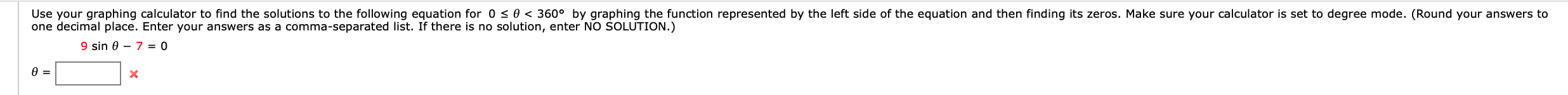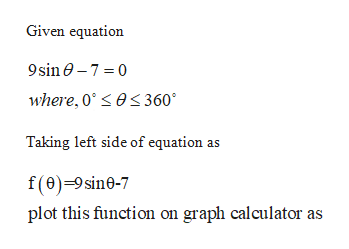# Use your graphing calculator to find the solutions to the following equation for 0

Questionhelp_outlineImage TranscriptioncloseUse your graphing calculator to find the solutions to the following equation for 0 <0 < 360° by graphing the function represented by the left side of the equation and then finding its zeros. Make sure your calculator is set to degree mode. (Round your answers to one decimal place. Enter your answers as a comma-separated list. If there is no solution, enter NO SOLUTION.) 9 sin 0 7 = 0 X fullscreen
check_circleExpert Solution
Step 1help_outlineImage TranscriptioncloseGiven equation 9sin0-7 0 where, 0es 360 Taking left side of equation as f(0)-9sine-7 plot this function on graph calculator as fullscreen
Step 2

### Want to see the full answer?

See Solution

#### Want to see this answer and more?

Solutions are written by subject experts who are available 24/7. Questions are typically answered within 1 hour*

See Solution
*Response times may vary by subject and question
Tagged in

### Trigonometry